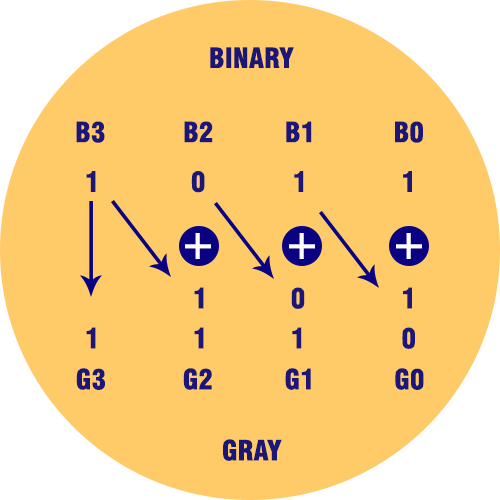﻿ Binary to Gray Code Converter Calculator | Calculators4U

# Binary to Gray Code Converter

Use our Binary to Gray code converter a free online calculator / conversion tool to convert binary value into gray code.

## Binary Codes

A symbolic representation of data / information is called code. The base or radix of the binary number is 2. Hence, it has two independent symbols. The symbols used are 0 and 1. A binary digit is called as a bit. A binary number consists of sequence of bits, each of which is either a 0 or 1. Each bit carries a weight based on its position relative to the binary point. The weight of each bit position is one power of 2 greater than the weight of the position to its immediate right. e. g. of binary number is 101011 which is equivalent to decimal number 43.

## Gray Codes

It is a non-weighted code; therefore, it is not a suitable for arithmetic operations. It is a cyclic code because successive code words in this code differ in one bit position only i.e. it is a unit distance code### Applications of Gray Code

In instrumentation and data acquisition systems, where linear or angular displacement is measured. In shaft encoders, input-output devices, A/D converters and the other peripheral equipment

### Binary to Gray Code Truth Table:

Decimal Binary Code (input) Gray Code (output)
0 0000 0000
1 0001 0001
2 0010 0011
3 0011 0010
4 0100 0110
5 0101 0111
6 0110 0101
7 0111 0100
8 1000 1100
9 1001 1101
10 1010 1111
11 1011 1110
12 1100 1010
13 1101 1011
14 1110 1001
15 1111 1000